# Heavy sphere, method of the

(diff) ← Older revision | Latest revision (diff) | Newer revision → (diff)

A method for solving a minimization problem for a differentiable function on a Euclidean space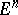. The method is based on considering the system of differential equations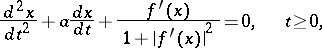(1)

which describes the movement of a material point over the surface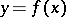in an attracting field directed in the negative direction of the-axis under the condition that the point may not leave the surface and that the attraction is proportional to the velocity;is de gradient ofat a pointand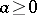is the attraction coefficient. Taking into account that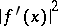is small in a neighbourhood of a stationary point, (1) is often replaced by the system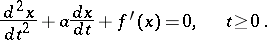(2)

Subject to certain assumptions onand under the initial conditionsit can be shown that the corresponding solutionof (1) or (2), as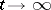, converges to some stationary point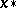of. Ifis a convex function, thenis a minimum point of it. Thus, the method of the heavy sphere is a particular case of the adjustment method. For the numerical solution of (1), or (2), one may, use, e.g., difference methods. In dependence on the choice of this difference method, discrete analogues of the method of the heavy sphere are obtained, including those for functions depending strongly on a few variables, the conjugate-gradient method, etc. (cf. Minimization methods for functions depending strongly on a few variables; Conjugate gradients, method of). The choice of the step of the difference method and of the quantitystrongly influences the rate of convergence of the method of the heavy sphere. Instead of (1), (2) other first- or second-order systems may be used (cf. ). In the problem of minimizing a functionunder the restrictions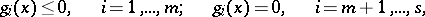the method of the heavy sphere is applied in combination with the method of penalty functions, Lagrange functions, etc. (cf. , , Penalty functions, method of; Lagrange function).

How to Cite This Entry:
Heavy sphere, method of the. Encyclopedia of Mathematics. URL: http://encyclopediaofmath.org/index.php?title=Heavy_sphere,_method_of_the&oldid=14643
This article was adapted from an original article by F.P. Vasil'ev (originator), which appeared in Encyclopedia of Mathematics - ISBN 1402006098. See original article